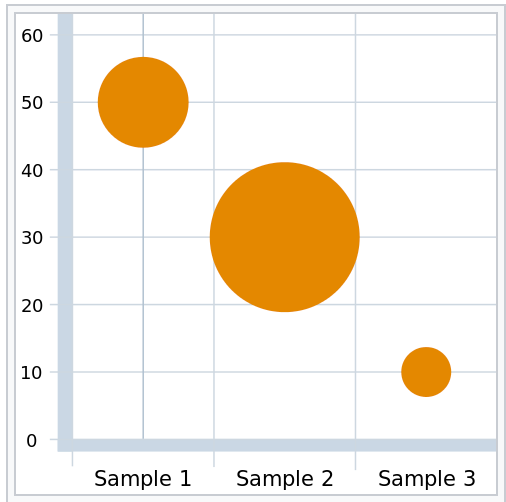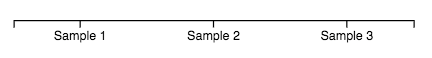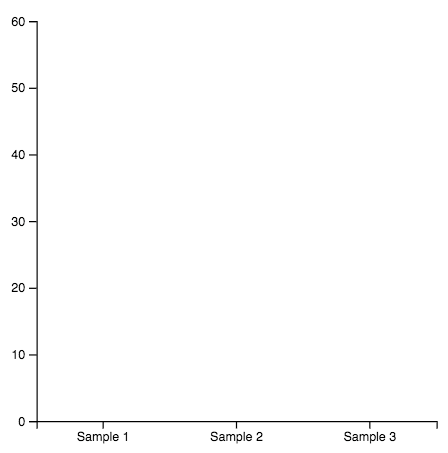# Bubble Chart in D3js

Understanding a chart with d3 v6

D3js is human readable sometimes, however in certain occasions you see a chart you want to create or just copy from somewhere else and

In this post, we will see a tutorial for basic bubble chart that may help you. To be honest, it is a tutorial also for myself. In the past few years, I have done like ten bubble charts and every time I start from scratch.

## Introduction

First question, what is Bubble Chart…

A scatterplot with variable dot size

from https://www.data-to-viz.com/ which is an amazing, fantastic and awesome resource that you should have a look if you are starting with DataViz (either has content with Python or R)

How a bubble chart looks likeFrom Wikipedia, a bubble chart show the relationship between three variables.

## Version 6

Why 6?

At this time is the latest stable version of d3. To me it is more compacted, you have more functions in its core and is easier to load its modules…

I leave you the link anyway for installing it in different environments

https://github.com/d3/d3#installing

But, I love npm so if you are like me …

`\$ npm i d3`

`index.js`

`import * as d3 from "d3";`

## Dataset

We are going to create something similar, now imagine you have a similar dataset

`const dataset = [{ type: "Sample 1", value: 50, radius: 7 },{ type: "Sample 2", value: 30, radius: 11 },{ type: "Sample 3", value: 10, radius: 3 }];`

But, first of all a couple of utility function in d3 to manipulate data

d3.extent to calculate min and max

`d3.extent(dataset, d => d.value) ==> [10, 50]`

However, our Y Axis starts at 0 so we can only use d3.max function and finish with 60.

`d3.max(dataset, d => d.value) + 10 ==> 60`

Moreover, we need an array with all the ordinal values so we can use map function from es6 or mapping from d3 that extracts the names.

`dataset.map(d => d.type) ==> ["Sample 1", "Sample 2", "Sample 3"]d3.map(dataset, d => d.type).keys() ==> ["Sample 1", "Sample 2", "Sample 3"]`

As you see d3 has some native functions over arrays that can be very useful.

## Svg

It is always the same, create a frame with margin, the width & height of the content, translating it correctly to visually /display correctly and so on and so forth…

`index.html`

`<div id="viz"></div>`

`index.js`

`const margin = { top: 40, right: 150, bottom: 60, left: 30 },width = 500 - margin.left - margin.right,height = 420 - margin.top - margin.bottom;const svg = d3.select("#viz").append("svg").attr("width", width + margin.left + margin.right).attr("height", height + margin.top + margin.bottom).append("g").attr("transform", "translate(" + margin.left + "," + margin.top + ")");`

## Axis and Scale

For X Axis we have to create and scale of discrete values equally distributed along the axis

`index.js`

`const types = dataset.map(d => d.type); ==> ["Sample 1", "Sample 2", "Sample 3"]// Add X scaleconst x = d3.scaleBand().domain(types).range([0, width]);// Add X axissvg.append("g").attr("transform", "translate(0," + height + ")").call(d3.axisBottom(x));`

At this point, you may wonder why I use scaleBand rather than scaleOrdinal ???

`scaleOrdinal` maps discrete values (specified by an array) to discrete values (also specified by an array)

And

`In scaleBand` domain is specified as an array of values (one value for each band) and the range is splitted automatically into n bands computing the positions and widths of them.

So basically with scaleBand we specify less and we get part of the job done automaticallyX Axis and X Scale that we have created with the code below

For understanding scales, another amazing resource is

https://www.d3indepth.com/scales/

For Y Axis we create a linear scale from 0 to the maximum value of values

`index.js`

`const yMax = d3.max(dataset, d => d.value) + 10 ==> 60// Add Y scaleconst y = d3.scaleLinear().domain([0, yMax]).range([height, 0]);// Add Y axissvg.append("g").call(d3.axisLeft(y));`Axis and Scales that we have created with the code below

## Bubbles

For Bubbles we create circles using radius value in dataset for size and positioning them with its type and value. Normally, things used to be more complex with a customized scale in radius and colorized bubbles.

`index.js`

`// Add bubblessvg.append("g").selectAll("bubble").data(dataset).enter().append("circle").attr("cx", d => x(d.type) + 50).attr("cy", d => y(d.value)).attr("r", d => d.radius).style("fill", "lightcyan").style("opacity", "0.7").attr("stroke", "black");`

## Conclusions

You can play with it, changing a lot of things and customize colors, axis, etc.

If you start with dataviz and d3js, try to go deeper understanding every thing you do. It is a hard task at the beginning, but there are a lot of resources and step by step you can do amazing things.